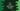# C++ program to print half pyramid or right angled triangle# Introduction :

In this C++ tutorial, we will learn how to print a half pyramid or right-angled triangle using numbers or using any character. The program will take the height of the triangle as an input from the user. With this example, you will learn how to read user inputs, how to print a message, how to use a separate function and how to use for loop in C++.

## C++ program to print right-angled triangle using numbers :

The complete C++ program looks like as below :

``````#include <iostream>
using namespace std;

void printHalfPyramid(int size)
{
for (int i = 1; i <= size; i++)
{
for (int j = 1; j <= i; j++)
{
cout << j << " ";
}
cout << "\n";
}
}

int main()
{
int size;

cout << "Enter the height of the triangle : " << endl; cin >> size;

printHalfPyramid(size);

return 0;
}``````

### Sample Output :

``````Enter the height of the triangle :
5
1
1 2
1 2 3
1 2 3 4
1 2 3 4 5

Enter the height of the triangle :
8
1
1 2
1 2 3
1 2 3 4
1 2 3 4 5
1 2 3 4 5 6
1 2 3 4 5 6 7
1 2 3 4 5 6 7 8 ``````

### How the program works :

In this program, we are using two for loops. The outer loop is used to point to the current row of the triangle i.e. if the value of i is 1, we are working on the first row, if its value is 2, we are working on the second row etc.

The inner loop is used to print the values for a specific row. We are using i for the outer loop and j for the inner loop. The inner loop will print from 1 to j. For example, for the third line, it will print from j = 1 to j = 3.

You can also modify the program to print the half pyramid using any character like below :

``````#include <iostream>
using namespace std;

void printHalfPyramid(int size, char c)
{
for (int i = 1; i <= size; i++)
{
for (int j = 1; j <= i; j++)
{
cout << c << " ";
}
cout << "\n";
}
}

int main()
{
int size;
char c;

cout << "Enter the height of the triangle : " << endl; cin >> size;

cout << "Enter the character : " << endl; cin >> c;

printHalfPyramid(size, c);

return 0;
}``````

It will print output like below :

``````Enter the height of the triangle :
5
Enter the character :
*
*
* *
* * *
* * * *
* * * * *

Enter the height of the triangle :
7
Enter the character :
\$
\$
\$ \$
\$ \$ \$
\$ \$ \$ \$
\$ \$ \$ \$ \$
\$ \$ \$ \$ \$ \$
\$ \$ \$ \$ \$ \$ \$ ``````

Just pass the character to the function and print this character instead of j.